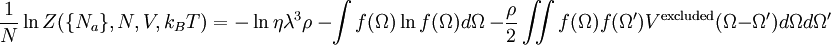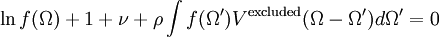# Onsager theory

The Onsager theory for the isotropic-nematic phase transition was developed by Lars Onsager (Ref. 1). In a 3-dimensional gas of hard rods there are two contributions to the entropy: a part due to translation and a part due to orientation. These two contributions are coupled. From the point of view of the translational component of the entropy, a parallel configuration of the rods is favored. This is because the excluded volume is effectively zero. However, from an orientational point of view a gas of perfectly aligned rods is a very low entropy configuration.

At very low densities the orientational term wins, i.e. there is very little gain in entropy to be made by reducing the excluded volume via parallel alignments. However, at very high densities it is clear that a perfectly aligned system is the most favorable. Thus at some point a transition must take place between the isotropic and nematic phases.

## Onsager equations$\frac{1}{N} \ln Z (\{N_a\}, N, V, k_BT) = -\ln \eta \lambda^3 \rho ~- \int f(\Omega) \ln f(\Omega) d\Omega ~- \frac{\rho}{2}\iint f(\Omega) f(\Omega') V^{\rm excluded} (\Omega - \Omega') d\Omega d\Omega'$

The second term on the right hand side is the contribution due to orientational entropy, and the third term is the effect of the excluded volume. One also has$\ln f(\Omega) +1 + \nu + \rho\int f(\Omega')V^{\rm excluded} (\Omega - \Omega') d\Omega' = 0$.

These are the Onsager equations.